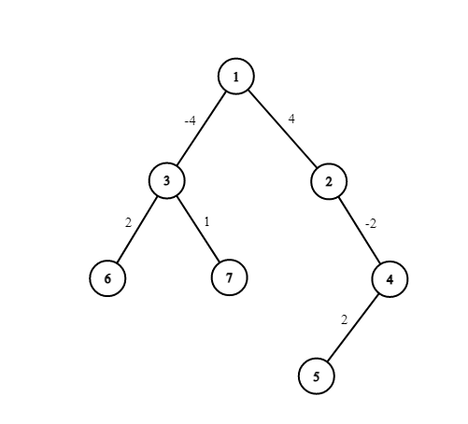Iftekhar_HakimIntra BUET Junior Program...
Limits 1s, 512 MB

“It's hard to say that I'd rather stay awake when I'm asleep
'Cause everything is never as it seems (when I fall asleep)”

Adam Young finally woke up. He discovered himself in Number-land. Number-land can be described as a rooted tree of $n$ junctions, where junction $1$ is the root. Each junction $i$ is connected with its parent junction $p_i$ by a road and an integer $v_i$ is written on that road.

Adam will start walking from junction $x$ with his board. Initially, his board has $0$ written on it. In every second, Adam can walk from his current junction to its parent junction by the road connecting them, or he can finish walking in his current junction. Of course, he cannot walk upward from the root junction. Whenever he crosses a road, he immediately adds the number written on that road to his board’s number.

Adam wants to maximize the final number written on his board after he finished his walking. But he will be sad if it is not divisible by his favorite number $K$. Help Adam finding the maximum number he can get on his board without being sad.

You have to find it for all $x$ $(1\le x \le n)$ independently, where $x$ is his starting junction.

## Input

First line of input will have number of junctions $n$ $(1\le n \le 10^5)$ and Adam’s favorite number $K$ $(1\le K \le 10^5)$.

Second line will have $n-1$ integers describing $p_i$ $(1\le p_i \le n)$ for $2\le i \le n$ respectively.

Third line will have $n-1$ integers describing $v_i$ $(-10^9 \le v_i \le 10^9)$ for $2\le i \le n$ respectively.

## Output

Output a single line consisting of $n$ integers. $i$-th integer will be the maximum number Adam can get on his board without being sad, if he starts walking from junction $i$.

## Sample

InputOutput
7 2
1 1 2 4 3 3
4 -4 -2 2 2 1

0 4 0 2 4 2 0This is the tree of sample input and $K=2$.

For junction $1$, Adam cannot move anywhere.

For junction $2$, $4$ and $5$, he will go upto junction $1$.

For junction $3$, it is optimal to move nowhere.

For junction $6$, he will move upto junction $3$.

For junction $7$, it is optimal to move nowhere. Note that, he cannot finish at junction $3$ starting from $7$, as his board’s number will not be divisible by $K$.GraphuDebug

### Submit